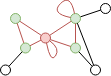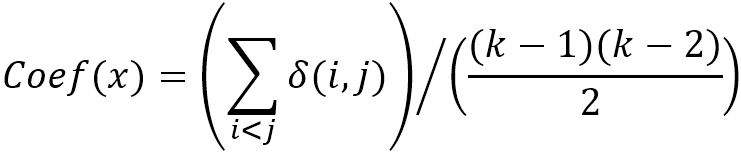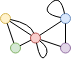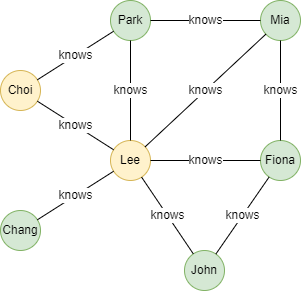# Change Nickname

Current Nickname:

Certifications

Certificate Issued at Valid until Serial No. File
Serial No. Valid until

Not having one? Apply now! >>>

Invoice

ProductName CreateTime ID Price File
ProductName CreateTime ID Price File

# Local Clustering Coefficient

## Overview

Local clustering coefficient of a node refers to the probability that the neighbours of the node are also connected.

Local clustering coefficient can be used to examine the tightness of the ego network of node. For example, in social network, it reveals how familiar the friends of one person are, which can help to distinguish the type of social group, such as relatives and friends, communities, agents, etc.

## Basic Concept

### Ego Network

Ego network is a subgraph formed by one center node (Ego) and all its 1-step neighbors (Alter). Please read Induced Subgraph algorithm for the introduction to subgraph.Specifying the red node in the graph above as ego, its ego network contains the red node, the green nodes and all the red edges.

### Local Clustering Coefficient

Local clustering coefficient of a node is obtained by dividing the number of its neighbor pairs which have edge in between by the number of all neighbor pairs:where `x` represents the node to be calculated, `i` and `j` are any two distinct neighbors in the ego network of `x`; `δ(i,j)` is 1 when there is edge between `i` and `j`, and 0 otherwise; `k` is the number of nodes in `x`'s ego network, `(k-1)(k-2)/2` is the number of node pairs of `i` and `j`.For the ego network of the red node in the graph above, only yellow-green and blue-purple node pairs have edge in between, thus the local clustering coefficient of the red node is `2 / 6 = 0.3333`.

## Special Case

### Lonely Node, Disconnected Graph

Lonely node does not connect with any other node, its local clustering coefficient is 0, and it does not participate in the calculation of any local clustering coefficient.

Nodes in one connected component must not participate in the calculation of the local clustering coefficient of nodes in other connected components.

### Self-loop Edge

Self-loop edge of a node does not increase the number of neighbors of the node.

### Directed Edge

For directed edges, the Local Clustering Coefficient algorithm ignores the direction of edges but calculates them as undirected edges.

## Results and Statistics

Take the 7-user social network graph below as an example, run the Local Clustering Coefficient algorithm:Algorithm results: Calculate local clustering coefficient for user Lee (UUID = 1) and Choi (UUID = 2), return `_id`, `centrality` or `_uuid`, `centrality` according to the execution method

_uuid _id centrality
1 Lee 0.26666668
2 Choi 1.0000000

Algorithm statistics: N/A

## Command and Configuration

• Command: `algo(clustering_coefficient)`
• Configurations for the parameter `params()`:
Name Type
Default
Specification
Description
ids / uuids []`_id` / []`_uuid` / / IDs or UUIDs of nodes to be calculated; all nodes to be calculated if not set
limit int -1 >=-1 Number of results to return; return all results if sets to -1 or not set
order string / ASC or DESC, case insensitive To sort the returned results; no sorting is applied if not set

Example: Calculate the local clustering coefficient of nodes UUID = 1,2,3

``````algo(clustering_coefficient).params({
uuids: [1,2,3]
}) as lcc
return lcc
``````

## Algorithm Execution

#### 1. File Writeback

Configuration Data in Each Row
filename `_id`,`centrality`

Example: Calculate the local clustering coefficient of user Lee and Choi, write the algorithm results back to file named centrality

``````algo(clustering_coefficient).params({
ids: ["Lee", "Choi"]
}).write({
file:{
filename: "centrality"
}
})
``````

#### 2. Property Writeback

Configuration Writeback Content Type Data Type
property `centrality` Node property `float`

Example: Calculate the local clustering coefficient of user Lee and Choi, write the algorithm results back to node property named lcc

``````algo(clustering_coefficient).params({
ids: ["Lee", "Choi"]
}).write({
db:{
property: "lcc"
}
})
``````

#### 3. Statistics Writeback

This algorithm has no statistics.

### Direct Return

Alias Ordinal
Type
Description Column Name
0 []perNode Node and its local clustering coefficient `_uuid`, `centrality`

Example: Calculate the local clustering coefficient of all nodes, define algorithm results as alias named results, and return the results

``````algo(clustering_coefficient).params() as results
return results
``````

### Streaming Return

Alias Ordinal
Type
Description Column Name
0 []perNode Node and its local clustering coefficient `_uuid`, `centrality`

Example: Calculate the local clustering coefficient of all nodes, define algorithm results as alias named results, and return the results with local clustering coefficient other than 0

``````algo(clustering_coefficient).params().stream() as results
where results.centrality > 0
return results
``````

### Real-time Statistics

This algorithm has no statistics.